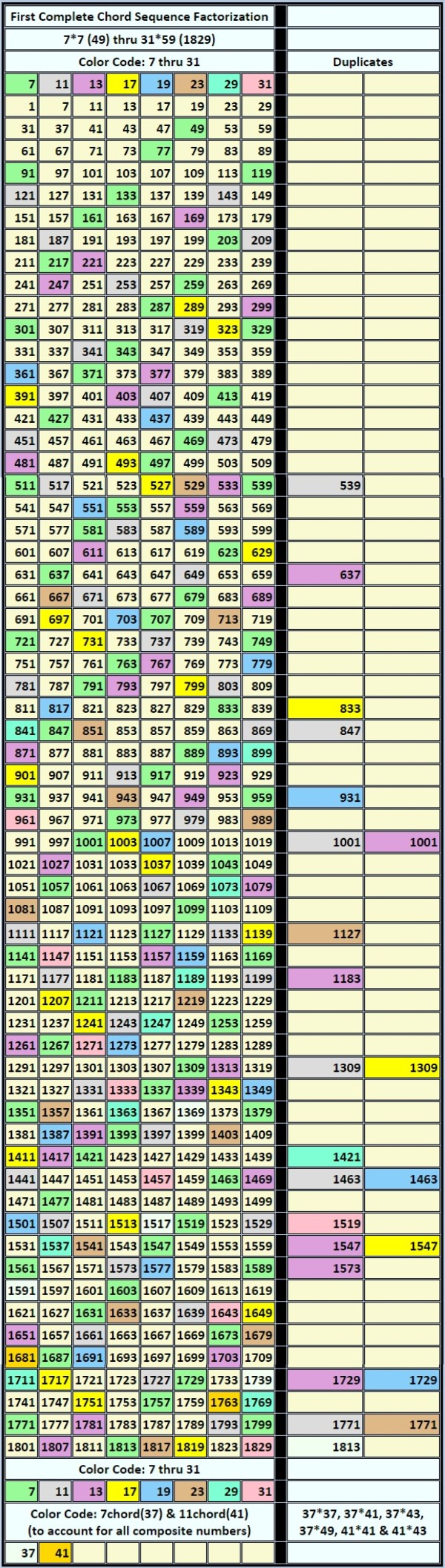# Chord Sequence Factorization Matrix

The table below, populated by natural numbers not divisible by 2, 3 and 5 between 1 and 1829 (arrayed in 8 columns) factorizes the chords (and partial chords) needed to account for all composite numbers in this range, i.e., 7chord(7), 11chord(11), 13chord(13), 17chord(17), 19chord(19), 23chord(23), 29chord(29), 1chord(31), 7chord(37) and 11chord(41). Upon completion of the factoring sequence all numbers not indexed to a product are prime (peach-colored boxes), except for the number 1.

The factorization involves multiplying each number in the array (except the number 1) times itself and every subsequent number ... n. For example, 7*7 = 49; 7*11 = 77 ... n; 11*11 = 121; 11*13 = 143 ... n; 13*13 = 169; 13*17 = 221 ... n, etc.

The "duplicates" (see the two right-hand columns) result when composite numbers in the sequence are factorized, e.g., 7*77 = 539 is equivalent to 11*49 = 539 (both 77 and 49 being composite numbers, and both expressions being equivalent to 7*7*11 = 539).